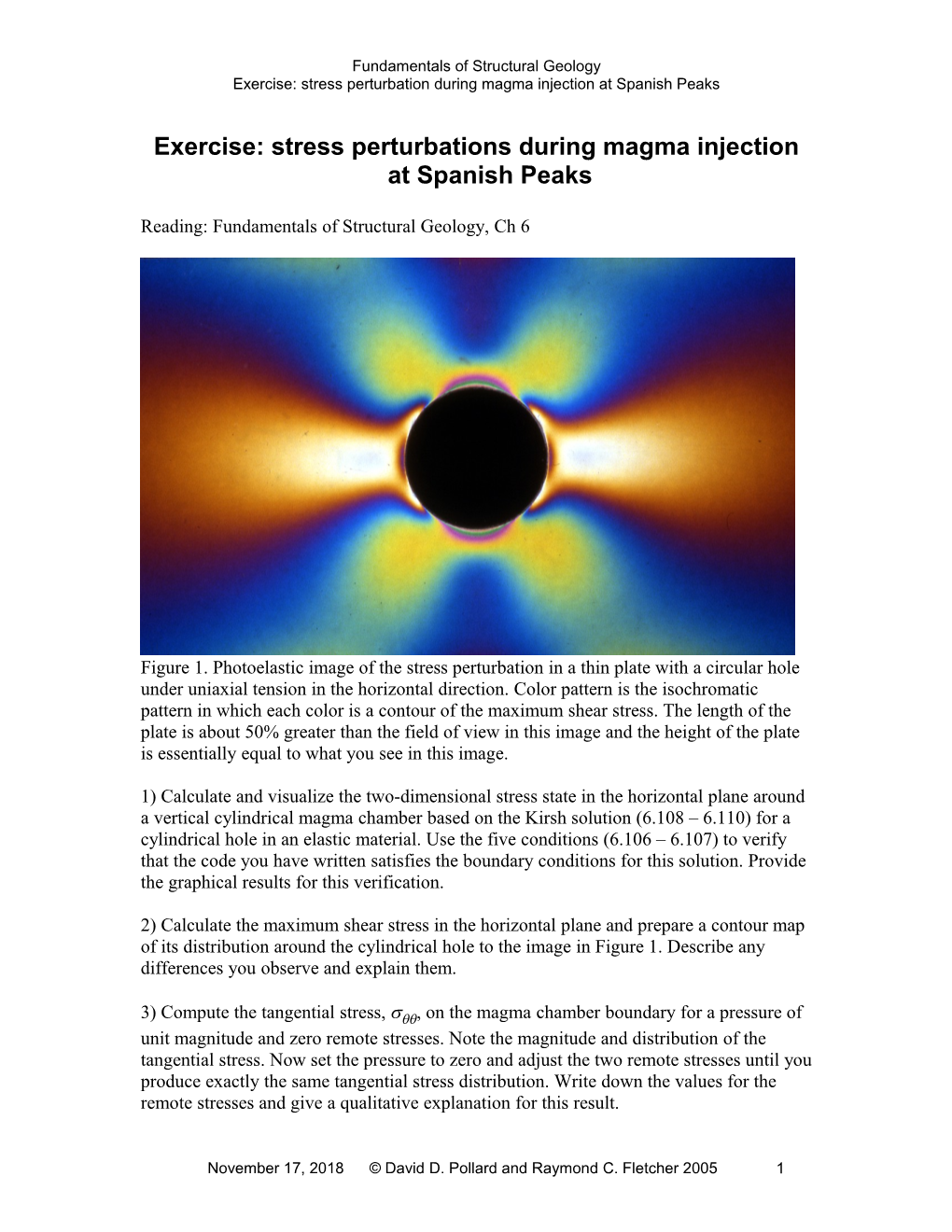# Exercise: Stress Perturbations During Magma Injection at Spanish PeaksFundamentals of Structural Geology

Exercise: stress perturbation during magma injection at Spanish Peaks

## Exercise: stress perturbations during magma injection at Spanish Peaks

Reading: Fundamentals of Structural Geology, Ch 6Figure 1. Photoelastic image of the stress perturbation in a thin plate with a circular hole under uniaxial tension in the horizontal direction. Color pattern is the isochromatic pattern in which each color is a contour of the maximum shear stress. The length of the plate is about 50% greater than the field of view in this image and the height of the plate is essentially equal to what you see in this image.

1) Calculate and visualize the two-dimensional stress state in the horizontal plane around a vertical cylindrical magma chamber based on the Kirsh solution (6.108 – 6.110) for a cylindrical hole in an elastic material. Use the five conditions (6.106 – 6.107) to verify that the code you have written satisfies the boundary conditions for this solution. Provide the graphical results for this verification.

2) Calculate the maximum shear stress in the horizontal plane and prepare a contour map of its distribution around the cylindrical hole to the image in Figure 1. Describe any differences you observe and explain them.

3) Compute the tangential stress, , on the magma chamber boundary for a pressure of unit magnitude and zero remote stresses. Note the magnitude and distribution of the tangential stress. Now set the pressure to zero and adjust the two remote stresses until you produce exactly the same tangential stress distribution. Write down the values for the remote stresses and give a qualitative explanation for this result.

4) Imagine that you have a very good tool for measuring stress states in rock in the field. Describe how you would design a field experiment to distinguish the two different loading conditions that lead to the same tangential stress distribution in part 2).

5) Apply only a remote stress of unit magnitude acting in the y coordinate direction. Calculate the stress concentration factors for the tangential stress along the hole boundary at  = 0° and  = 90°. Explain how these differ from the stress concentration factors caused by a remote stress of the same magnitude acting only in the x direction? What does this result tell you about the symmetry of the stress state?

6) Consider a vertical cylindrical magma chamber at 4 km depth with horizontal remote compressive principal stresses of magnitude SH = 125 MPa in x and Sh = 100 MPa in y. What is the minimum value of magma pressure, P, that produces a stress state necessary for a dike to begin propagating away from the chamber. What is the minimum value of magma pressure that produces tension everywhere along the wall of the chamber? Would you expect the magma pressure ever to achieve this value? Explain your reasoning.

7) Continue with the same remote loading conditions as in part 5) and use an internal pressure of magnitude P = 175 MPa. If a magma chamber is 5 km in radius, how far from the chamber would you expect to see significant (>10%) perturbations of the remote field? Plot contour maps of the mean normal stress and the maximum shear stress to address this question. Use this information to define the size of the region of stress perturbationdue todevelopment of the magma chamber.

8) Anderson (Anderson, 1972) proposed that igneous dikes propagate perpendicular to the local least compressive stress. Figure 2 shows one of the dikes at Spanish Peaks.Figure 2. WestSpanishPeak (background) and one of the radial dikes (foreground) (Muller and Pollard, 1977).

Plot a map of the stress trajectories in the horizontal plane around the vertical cylindrical magma chamber. A plot of such trajectories is compared to the traces of dikes in the Spanish Peaks region in Figure 3. Set the magnitude of the magma pressure to P = 175 MPa. Add remote compressive stresses in the range 0 to 200 MPa and determine what remote states produce a purely radial pattern of trajectories. What general conclusions can one draw from a map pattern of dikes that is almost purely radial?

9) Set the magnitude of the magma pressure to P = 175 MPa. Vary the difference between the two remote principal stresses and qualitatively describe the effect on the stress trajectory pattern. What can one learn about the state of stress from the orientations of dikes at significant distances from the magma chamber?Figure 3. Map of Spanish Peaks region of southeastern Colorado with traces of dikes (dotted curves) and trajectories of greatest compressive horizontal stress (short solid lines) shown in alternating vertical strips (Muller and Pollard, 1977).

1

November 25, 2018© David D. Pollard and Raymond C. Fletcher 2005